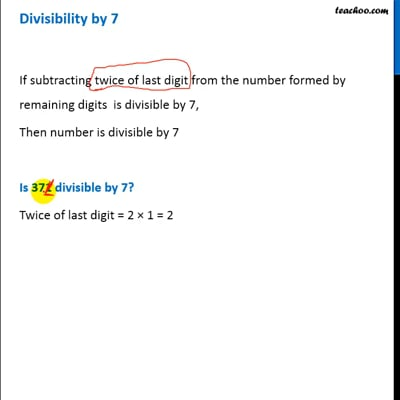Divisibility Tests - All

Chapter 3 Class 6 Playing with Numbers
Concept wise

If subtracting twice of last digit from the number formed by  remaining digits  is divisible by 7,

Then number is divisible by 7

#### Is 371 divisible by 7?

Twice of last digit = 2 × 1 = 2

Remaining digits = 37

Subtraction = 37 – 2 = 35

Since 35 is divisible by 7

∴ 371 is divisible by 7

#### Is 434 divisible by 7?

Twice of last digit = 2 × 4 = 8

Remaining digits = 43

Subtraction = 43 – 8 = 35

Since 35 is divisible by 7

∴ 434 is divisible by 7

#### Is 672 divisible by 7?

Twice of last digit = 2 × 2 = 4

Remaining digits = 67

Subtraction = 67 – 4 = 63

Since 63 is divisible by 7

∴ 672 is divisible by 7

#### Is 105 divisible by 7?

Twice of last digit = 2 × 5 = 10

Remaining digits = 10

Subtraction = 10 – 10 = 0

Since 0 is divisible by 7

∴ 105 is divisible by 7

#### Is 905 divisible by 7?

Twice of last digit = 2 × 5 = 10

Remaining digits = 90

Subtraction = 90 – 10 = 80

Since 80 is not divisible by 7

∴ 905 is not divisible by 7This video is only available for Teachoo black users

Introducing your new favourite teacher - Teachoo Black, at only ₹83 per month